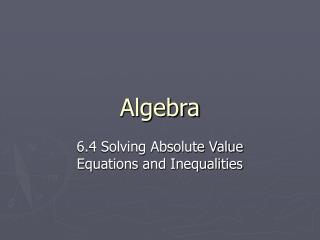DownloadDownload PresentationAlgebra

# Algebra

Télécharger la présentation## Algebra

- - - - - - - - - - - - - - - - - - - - - - - - - - - E N D - - - - - - - - - - - - - - - - - - - - - - - - - - -
##### Presentation Transcript

1. Algebra 6.4 Solving Absolute Value Equations and Inequalities

2. Steps to Solve an Absolute Value Equation • Isolate the absolute value expression • Drop the absolute value and create a positive and a negative equation • Solve each

3. Solving an Absolute Value Equation • Solve lx – 4l = 8 x – 4 = 8 or x – 4 = -8 +4 +4 +4 +4 x = 12 or x = -4 Mentally check your answers.

4. Solving an Absolute Value Equation • Solve l5x + 1l + 3 = 14 Isolate the absolute value expression. l5x + 1l = 11 5x + 1 = 11 or 5x + 1 = -11 -1 -1 -1 -1 5x = 10 or 5x = -12 x = 2 or x = - 2 and 2/5 Mentally check your answers. -3 -3 5 5

5. 1) lxl ≤ 2 (The distance from 0 is less than or equal to 2) 2) lxl > 3 (The distance from 0 is greater than 3) 3) lx + 2l ≥ 1 4) lx – 3l ≤ 2 Investigating Absolute-Value InequalitiesSee if each number is a solution as it appears. If it is, fill it in. . . . . . . . . . . . . . . . -5 -4 -3 -2 -1 0 1 2 3 4 5 -5 -4 -3 -2 -1 0 1 2 3 4 5 x ≤ 2 and x ≥ -2 or . . . . . . . . . . . . . -5 -4 -3 -2 -1 0 1 2 3 4 5 -4 -3 -2 -1 0 1 2 3 4 5 6 x > 3 or x < -3 WHY? and Absolute Value > or ≥ is an or inequality Absolute Value < or ≤ is an and inequality

6. Steps to Solve an Absolute Value Inequality • Isolate the Absolute Value Expression • Drop the absolute value and create a positive inequality and a negative inequality (flip the sign) • Solve each inequality separately • <, ≤ is an and inequality >, ≥ is an or inequality 5) Graph

7. Let’s Try! Solve lx + 6l < 8 x + 6 < 8 and x + 6 > -8 -6 -6 -6 -6 x < 2 and x > -14 0 2 -14

8. You Try! Solve l2x - 4l ≥ 2 2x - 4 ≥ 2 or 2x - 4 ≤ -2 +4 +4 +4 +4 2x ≥ 6 or 2x ≤ 2 x ≥ 3 or x ≤ 1 1 2 3 0

9. Let’s Try! Solve l2x + 1l - 3 ≥ 6 l2x + 1l – 3 ≥ 6 +3 +3 l2x + 1l ≥ 9 2x + 1 ≥ 9 or 2x + 1 ≤ -9 -1 -1 -1 -1 2x ≥ 8 or 2x ≤ -10 x ≥ 4 or x ≤ -5 -5 4 0

10. You Try! Solve l3x - 3l + 4 < 10 l3x - 3l + 4 < 10 -4 -4 l3x - 3l < 6 3x - 3 < 6 and 3x - 3 > -6 +3 +3 +3 +3 3x < 9 and 3x > -3 x < 3 and x > -1 -1 3 0

11. HW • P. 356-358 (19-59 Odd, 77-83 Odd)